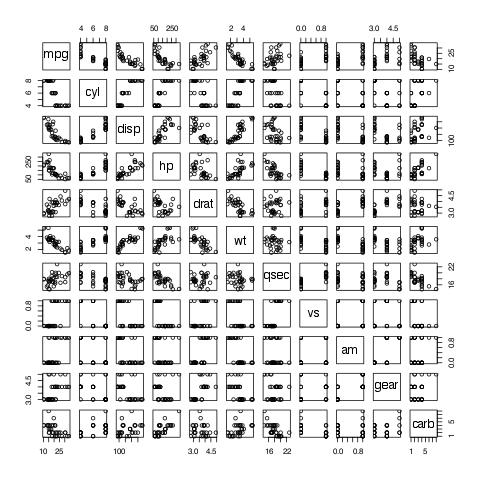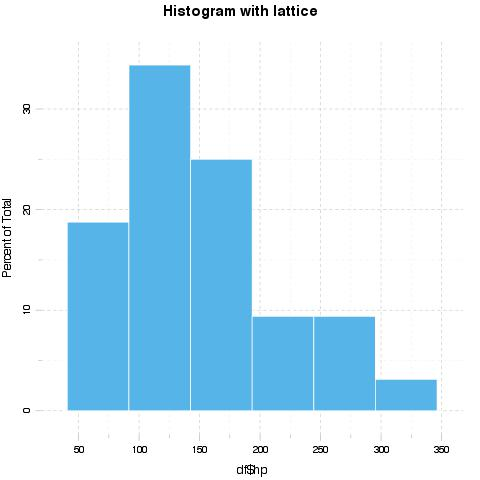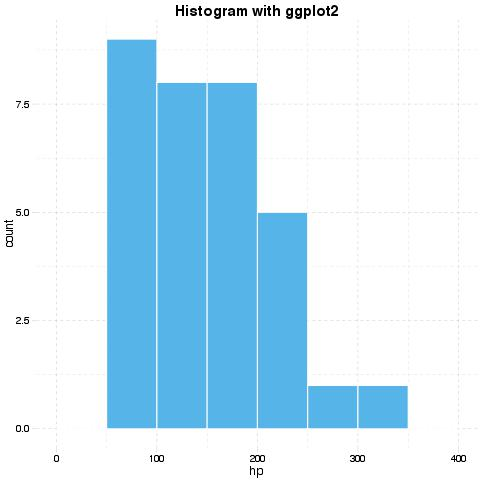# Capturing evaluation information with evals In pander: An R 'Pandoc' Writer

```knitr::opts_chunk\$set(collapse = TRUE, comment = "#>")
library(pander)
library(logger)
evalsOptions('graph.name', 'test')
evalsOptions('graph.dir', 'my_plots')
evalsOptions('graph.output', 'jpg')
```

`evals` is aimed at collecting as much information as possible while evaluating R code. It can evaluate a character vector of R expressions, and it returns a list of information captured while running them:

• `src` holds the R expression,
• `result` contains the raw R object as-is,
• `output` represents how the R object is printed to the standard output,
• `type` is the class of the returned R object,
• `msg` is a list of messages captured while evaluating the R expression. Among other messages, warnings/errors will appear here.
• `stdout` contains what, if anything, was written to the standard output.

Besides capturing evaluation information, `evals` is able to automatically identify whether an R expression is returning anything to a graphical device, and can save the resulting image in a variety of file formats.

Another interesting `evals` feature is caching the results of evaluated expressions. Read the caching section for more details.

`evals` has a large number of options, which allow users to customize the call exactly as needed. Here we will focus on the most useful features, but the full list of options, with explanations, can be viewed by calling `?evalsOptions`. Also `evals` support permanent options that will persist for all calls to `evals`, this can be achieved by calling `evalsOptions`.

Let's start with a basic example by evaluating `1:10` and collecting all information about it:

```evals('1:10')
```

Not all the information might be useful, so `evals` makes it is possible to capture only some of the information, by specifying the `output` parameter:

```evals('1:10', output = c('result', 'output'))
```

One of the neat features of `evals` that it catches errors/warnings without interrupting the evaluation and saves them.

```evals('x')[]\$msg
evals('as.numeric("1.1a")')[]\$msg
```

## Graphs and Graphical Options

As mentioned before, `evals` captures the output to graphical devices and saves it:

```evals('plot(mtcars)')[]\$result
```You can specify the output directory using the `graph.dir` parameter, and the output type using the `graph.output` parameter. Currently, it could be any of `grDevices`: `png`, `bmp`,`jpeg`,`jpg`, `tiff`, `svg`, or `pdf`.

```evals('plot(mtcars)', graph.dir = 'my_plots', graph.output = 'jpg')[]\$result
```

Moreover, `evals` provides facilities to:

• save the environments in which plots were generated
• save the plot via `recordPlot` to distinct files with `recodplot` extension
• save the raw R object returned (usually with `lattice` or `ggplot2`) while generating the plot to distinct files with `RDS` extension

### Style unification

`evals` provides very powerful facilities to unify the styling of images produced by different packages, like `ggplot2` and `lattice`.

Let's prepare the data for plotting:

```## generating dataset
set.seed(1)
df <- mtcars[, c('hp', 'wt')]
df\$factor <- sample(c('Foo', 'Bar', 'Foo bar'), size = nrow(df), replace = TRUE)
df\$factor2 <- sample(c('Foo', 'Bar', 'Foo bar'), size = nrow(df), replace = TRUE)
df\$time <- 1:nrow(df)
```
```## loading packages
require(ggplot2, quietly = TRUE)
require(lattice, quietly = TRUE)
```

Now let's plot the histograms:

```evalsOptions('graph.unify', TRUE)
evals('histogram(df\$hp, main = "Histogram with lattice")')[]\$result
evals('ggplot(df) + geom_histogram(aes(x = hp), binwidth = 50) + ggtitle("Histogram with ggplot2")')[]\$result
evalsOptions('graph.unify', FALSE)
```Options for unification can be set with `panderOptions`. For example:

```panderOptions('graph.fontfamily', "Comic Sans MS")
panderOptions('graph.fontsize', 18)
panderOptions('graph.fontcolor', 'blue')
panderOptions('graph.grid.color', 'blue')
panderOptions('graph.axis.angle', 3)
panderOptions('graph.boxes', T)
panderOptions('graph.legend.position', 'top')
panderOptions('graph.colors', rainbow(5))
panderOptions('graph.grid', FALSE)
panderOptions('graph.symbol', 22)
```

More information and examples on style unification can be obtained by `Pandoc.brew`ing the tutorial available here.

## Logging

To make execution and debugging easier to understand, `evals` provides logging with the `log` parameter. Logging in `evals` relies on the `logger` package, which provides a logging API similar to `log4j`. Basic example:

```x <- evals('1:10', log = 'foo')
```

`logger`'s thresholds range from most verbose to least verbose: `TRACE`, `DEBUG`, `INFO`, `WARN`, `ERROR`, `FATAL`. The threshold defaults to `INFO`, which will hide some unessential information. To permanently set the threshold for logger use `log_threshold`:

```evalsOptions('log', 'evals')
log_threshold(TRACE, namespace = 'evals')
x <- evals('1:10', cache.time = 0)
```

`logger` also provides a very useful ability to write logs to files instead of printing them to the prompt:

```t <- tempfile()
log_appender(appender_file(t), namespace = 'evals')
x <- evals('1:10', log = 'evals')
# revert back to console
log_appender(appender_stdout, namespace = 'evals')
```

## Result Caching

`evals` is uses a custom caching algorithm to cache the results of evaluated R expressions.

### How it works

• All R code passed to `evals` is split into single expressions and parsed.
• For each R expression (function call, assignment, etc.), `evals` extracts symbols in a separate list in `getCallParts`. This list describes the unique structure and the content of the passed R expressions
• A hash is computed for each list element and cached in `pander`'s local environments. This is useful if you are using large data frames; otherwise, the caching algorithm would have to compute the hash for the same data frame each time it's touched! This way the hash is recomputed only if the R object with the given name is changed.
• The list of such R objects is serialized, then an SHA-1 hash is computed, taking into consideration `panderOptions` and `evalsOptions`, which all together is unique and there is no real risk of collision.
• If `evals` can find the cached results in the appropriate environment (if `cache.mode set` to environment) or in a file named to the computed hash (if `cache.mode` set to `disk`), then it is returned on the spot. The objects modified/created by the cached code are also updated.
• Otherwise the call is evaluated and the results and the modified R objects of the environment are optionally saved to cache (e.g. if `cache` is active and if the evaluation `proc.time()` > `cache.time` parameter). Cached results are saved in `cached.results` in `pander`'s namespace. `evals` also remembers if R expressions change the evaluation environment (for example assignments) and saves such changes in `cached.environemnts` in `pander`'s namespace.

### Examples

We will set `cache.time` to 0, to cache all expressions regardless of time they took to evaluate. We will also use the logging facilites described above to simplify the understanding of how caching works.

```evalsOptions('cache.time', 0)
evalsOptions('log', 'evals')
log_threshold(TRACE, 'evals')
```

```system.time(evals('1:1e5'))
system.time(evals('1:1e5'))
```

Results cached by `evals` can be stored in an environment in current `R` session or permanently on disk by setting the `cache.mode` parameter appropriately.

```res <- evals('1:1e5', cache.mode = 'disk', cache.dir = 'cachedir')
list.files('cachedir')
```

Since the hash for caching is computed based on the structure and content of the R commands, instead of the variable names or R expressions, `evals` is able to achieve great results:

```x <- mtcars\$hp
y <- 1e3
system.time(evals('sapply(rep(x, y), mean)'))
```

Let us create some custom functions and variables, which are not identical to the above call:

```f <- sapply
g <- rep
h <- mean
X <- mtcars\$hp * 1
Y <- 1000
system.time(evals('f(g(X, Y), h)'))
```

Another important feature of `evals` is that it notes changes in the evaluation environment. For example:

```x <- 1
res <- evals('x <- 1:10;')
```

`x <- 1:10` will be cached; if the same assignment occurs again we won't need to evaluate it. But what about the change of `x` when we get the result from the cache? `evals` takes care of that.

So in the following example we can see that `x <- 1:10` is not evaluated, but retrieved from cache with the change to `x` in the environment.

```evals('x <- 1:10; x')[]\$result
```

Also `evals` is able to cache output to graphical devices produced during evaluation:

```system.time(evals('plot(mtcars)'))
system.time(evals('plot(mtcars)'))
```
```unlink('cachedir', recursive = TRUE, force = TRUE)
unlink('my_plots', recursive = TRUE, force = TRUE)
```

## Try the pander package in your browser

Any scripts or data that you put into this service are public.

pander documentation built on June 13, 2021, 9:06 a.m.